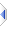# sum / product

 Definition: atom res = sum(object x, zlr=0) -- or -- atom res = product(object x, zlr=1) Description: Add or multiply together all elements of x, however deeply nested. x: atom or sequence of items to add or multiply together zlr: atom or length 1 sequence, the overrideable zero length result for {}. Comments: This function may be applied to an atom or all elements of a sequence. Note that unlike most of the other math functions there are no separate sq_sum or sq_product routines. The zlr (zero length result) parameter is only used when x is {}, an empty sequence. You might, for instance, rely on sum({},1) to award one bonus point for "no faults", or product({},0) to order zero tons of concrete when there is no foundation, instead of the mathematically correct defaults which are the other way around. If zlr is a sequence of length 1, containing a single atom, it is applied to any sum/product calls on (empty) nested subsequences, whereas if zlr is an atom it is applied at the top-level only, and any recursive calls get the standard 0/1 defaults. A fatal error occurs if zlr is not an atom or a length 1 sequence containing a single atom. Example: ```atom s = sum({1,2,3,4}), -- s is 10 p = product({1,2,3,4}) -- p is 24 ``` Implementation: See builtins\psum.e (an autoinclude) for details of the actual implementation.